Home > INT3 > Chapter 7 > Lesson 7.2.3 > Problem7-86

7-86.
1. What is the inverse function of each of the following functions? Use any method to write an equation for the inverse function. Be sure to restrict domains as necessary. 7-86 HW eTool (Desmos) Desmos Accessibility Homework Help ✎

1. f(x) = x2 + 3

2. f(x) =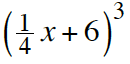3. f(x) =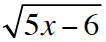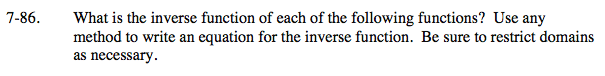Use the eTool below to graph the function and its inverse.
Click the link at right for the full version of the eTool: Draw Inverse eTool
Domain restrictions can be added to a function by using brackets, for example {x > 1}. Make sure both of the graphs are functions.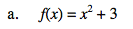This function squares the input then adds 3. How can this be undone? Use the eTool to check your answer.

Click the link below for the answer eTool: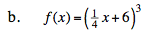Let f(x) = y. Interchange x and y. Solve for y in the new equation.

$x = \left( \frac{1}{4}y+6\right)^3$

$\sqrt{x}=\frac{1}{4}y+6$

$\sqrt{x}-6=\frac{1}{4}y$

$f^{-1} = 4(\sqrt{x}-6)$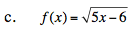To undo square rooting, square both sides of the equation.

$f^{-1}(x)=\frac{x^2+6}{5}$